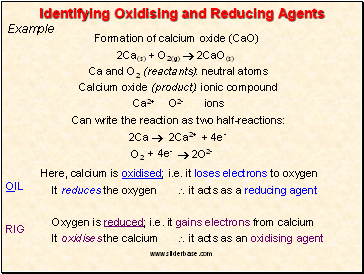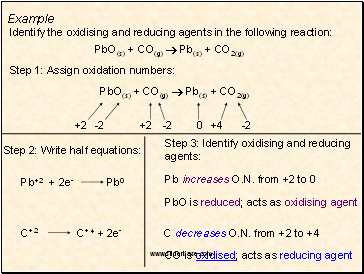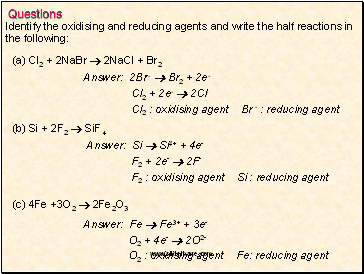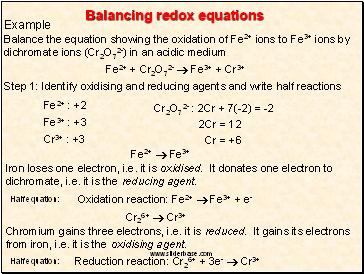# Balancing Chemical Equations. Balancing redox equationsPage 3

#### WATCH ALL SLIDES

OIL RIG

Oxidation can be defined (in terms of electon transfer) as the loss of electrons from a species

Reduction can be defined as the gain of electrons by a species

An oxidising agent (or electron acceptor) must itself be reduced

A reducing agent (or electron donor) must itself be oxidised

The action of both oxidising and reducing agents can be represented in the form of a half-equation

Slide 11## Identifying Oxidising and Reducing Agents

Example

Formation of calcium oxide (CaO)

2Ca(s) + O2(g)  2CaO(s)

Ca and O2 (reactants): neutral atoms

Calcium oxide (product): ionic compound

Ca2+ O2- ions

Can write the reaction as two half-reactions:

2Ca  2Ca2+

O2  2O2-

Here, calcium is oxidised; i.e. it loses electrons to oxygen

It reduces the oxygen

 it acts as a reducing agent

Oxygen is reduced; i.e. it gains electrons from calcium

It oxidises the calcium

 it acts as an oxidising agent

+ 4e-

+ 4e-

OIL

RIG

Slide 12Example

PbO(s) + CO(g)  Pb(s) + CO2(g)

Identify the oxidising and reducing agents in the following reaction:

+2

-2

+2

-2

0

+4

-2

Pb increases O.N. from +2 to 0

PbO is reduced; acts as oxidising agent

C decreases O.N. from +2 to +4

CO is oxidised; acts as reducing agent

Step 1: Assign oxidation numbers:

PbO(s) + CO(g)  Pb(s) + CO2(g)

Step 2: Write half equations:

Step 3: Identify oxidising and reducing agents:

Slide 13Questions

Identify the oxidising and reducing agents and write the half reactions in

the following:

(c) 4Fe +3O2  2Fe2O3

Answer: Fe  Fe3+ + 3e-

O2 + 4e-  2O2-

O2 : oxidising agent Fe: reducing agent

(b) Si + 2F2  SiF4

Answer: Si  Si4+ + 4e-

F2 + 2e-  2F-

F2 : oxidising agent Si : reducing agent

(a) Cl2 + 2NaBr  2NaCl + Br2

Answer: 2Br--  Br2 + 2e-

Cl2 + 2e-  2Cl-

Cl2 : oxidising agent Br - : reducing agent

Slide 14## Balancing redox equations

Example

Balance the equation showing the oxidation of Fe2+ ions to Fe3+ ions by dichromate ions (Cr2O72-) in an acidic medium

Fe2+ + Cr2O72-  Fe3+ + Cr3+

Step 1: Identify oxidising and reducing agents and write half reactions

Fe2+ : +2

Cr2O72- : 2Cr + 7(-2) = -2

2Cr = 12

Cr = +6

Fe3+ : +3

Cr3+ : +3

Fe2+  Fe3+

Iron loses one electron, i.e. it is oxidised. It donates one electron to dichromate, i.e. it is the reducing agent.

Go to page:
1  2  3  4  5  6  7  8